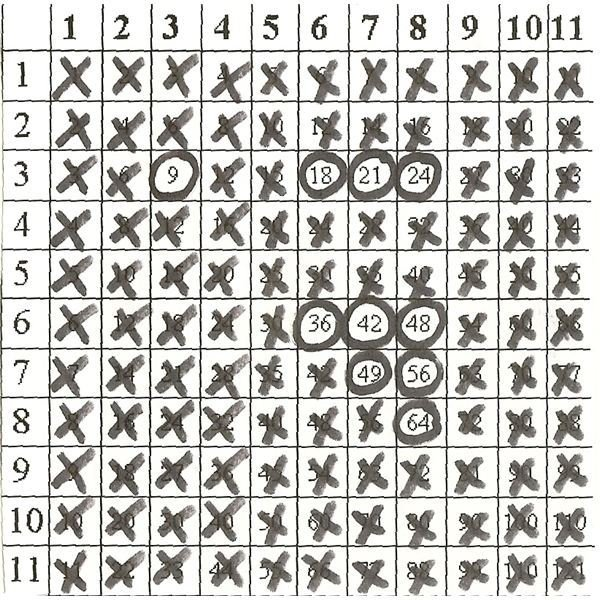# Multiplication Facts - Tips, Rules and Tricks to Help You Learn

Page content

## Basic Concepts First

Memorizing the entire Multiplication Table can seem quite overwhelming at first. The key to learning your multiplication facts is to break the process down into manageable lessons. This is done through a series of rules or “tricks” that can be learned. Once these have been mastered, you will see that it is only necessary to memorize ten multiplication facts! First, however, there are several key concepts that must be understood. [caption id=“attachment_130454” align=“aligncenter” width=“640”]Multiplication can be performed with basic addition and subtraction[/caption]

• The first is that multiplication is simply a fast way of joining groups of equal size through repeated addition. Let’s look at a problem together:

Sarah has 4 boxes of crayons. There are 3 crayons in each box. How many crayons does Sarah have altogether? This problem can be solved through repeated addition:

3+3+3+3 = 12

A shortened version of this would be to use the multiplication sentence:

4 x 3 = 12

• The second concept that must be understood is what each number in the multiplication problem represents. Let’s look at that same problem again:

Sarah has 4 boxes of crayons. There are 3 crayons in each box. How many crayons does Sarah have altogether?

4 x 3 = 12

In this case, the (4) represents the number of groups in the problem. (There were 4 boxes.) The (3) represents how many objects/items were in each group. (There were 3 crayons in each box.)

• The third concept that will help you with learning your multiplication facts is the Commutative Property of multiplication. This states that when two numbers are multiplied together, the product (or answer) is the same regardless of the order of the numbers. For instance:

3 x 2 = 6

2 x 3 = 6

3 x 2 = 2 x 3

## Rules and Tricks

Now that you understand the basic concepts of multiplication, it is time to look at the repeated patterns which can be found in the multiplication table…and ultimately used to solve times problems. These rules or tricks can be broken down into related fact families to help you learn your multiplication facts:

• 0’s: Anything times 0 equals 0 (0 x 2 = 0).
• 1’s: Anything times 1 equals itself (4 x 1 = 4).
• 2’s: Any number times 2 is doubled (4 x 2 = 8) / (4 + 4 = 8).
• 4’s: Any number times 4 is doubled once and then doubled again (6 x 4 = 24) / (6 + 6 = 12…12 + 12 = 24).
• 5’s: Use what you know about skip counting by 5 when multiplying by 5 (3 x 5 = 15) / (5, 10 15).
• 9’s: When multiplying by 9, use your addition and subtraction. Subtract one from the number you are multiplying. Then, think about the number you would add to it to equal 9. (4 x 9 = 36) / (4 -1 = 3…3 + 6 = 9).
• 10’s: Add a zero to any number that you multiply by 10. (6 x 10 = 60).
• 11’s: Think of 11 as two ones. Any number times 11 is that number times one and then that number times one again. (3 x 11 = 33) / (3 x 1 = 3…3 x 1 = 3).

## Only 10 Problems Are Left to Memorize!Unfortunately, some problems simply do no fit into the patterns found in the times table. These problems must be memorized. The good news is that once you incorporate the Commutative Property of Multiplication, you are left with 10 problems instead of 20! The circled problems in the Multiplication Table shown represent the 10 problems that you are best off memorizing. Take a look at Math-drills.com for handy worksheets you can print off and quiz your students on. By employing these simple tips and tricks, you will quickly be on your way to learning your multiplication facts! Congratulations!

• The multiplication table image and information offered in this article are based on the author’s experience as a classroom teacher, peer instructor and author of local math curriculum standards
• Image by Gerd Altmann from Pixabay